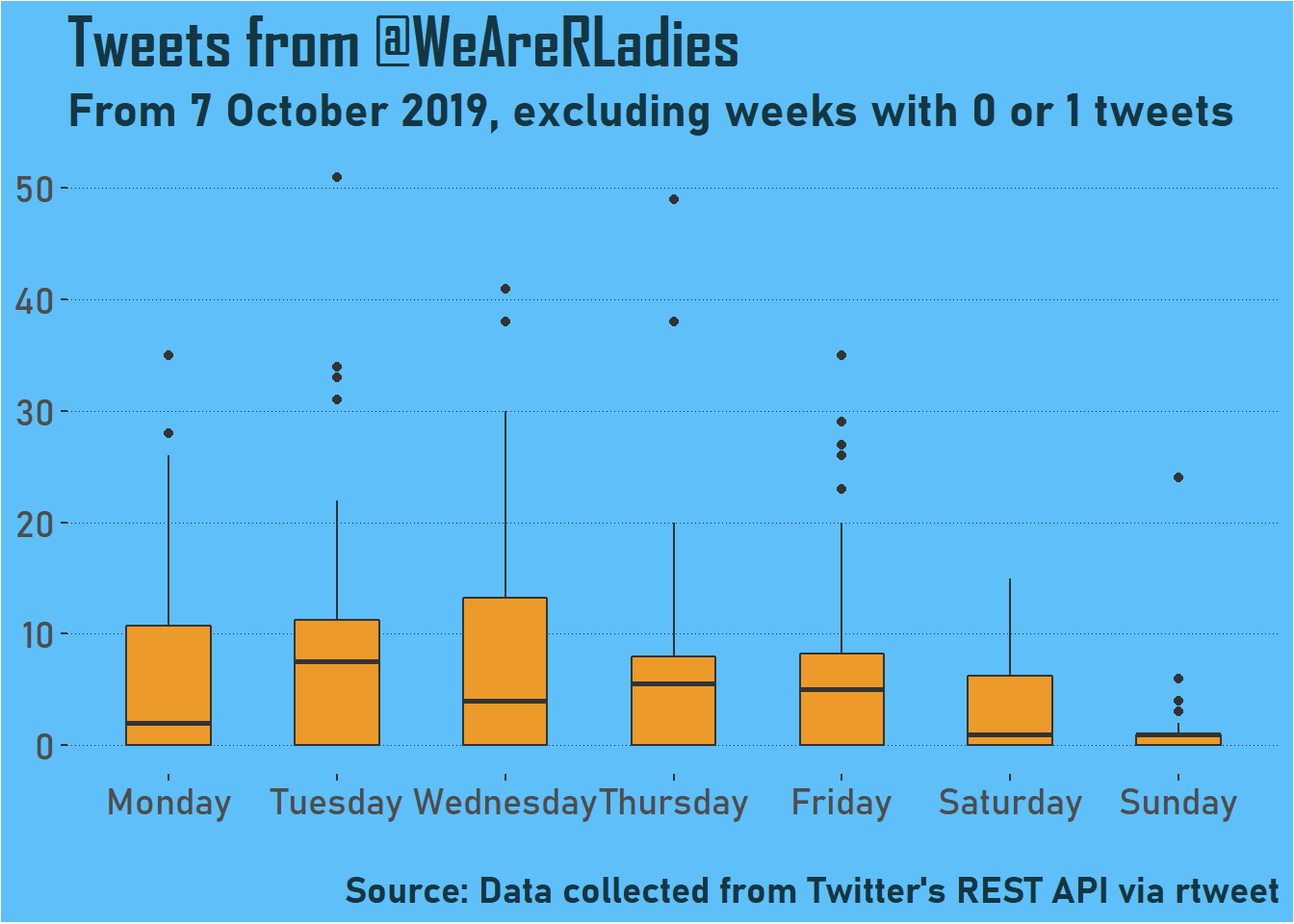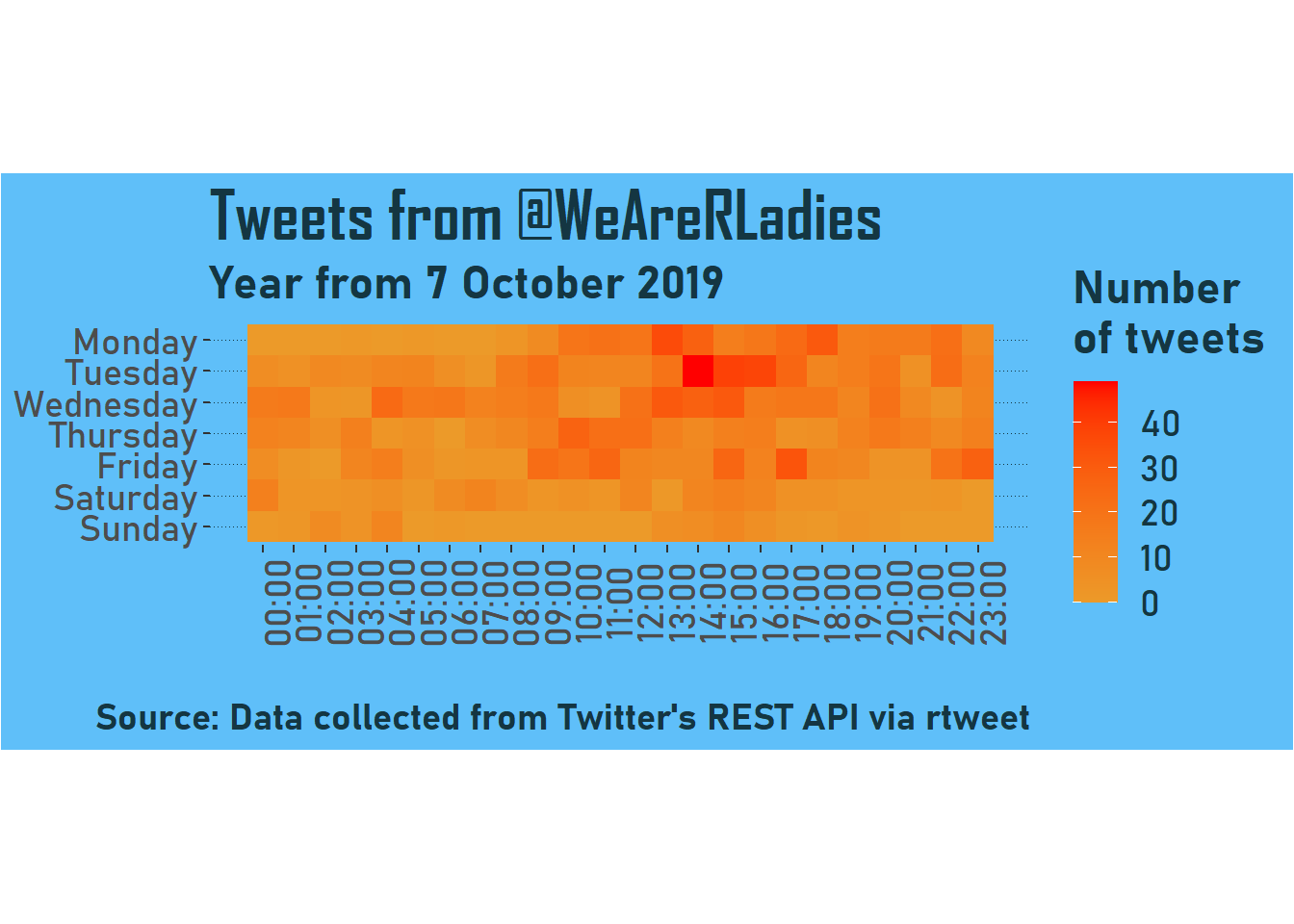# Tweet timing

I explored how often curators of @WeAreRLadies tweet in my last post. In this post, I go a little further with this analysis and explore how activity changes depending on the day of the week and time of day.

I’m using the same data that I retrieved using `rtweet` previously. I’m also going to use the same plot theme.

``````library(data.table)
library(ggplot2)
library(lubridate)

plot_theme <- theme(
plot.background = element_rect(fill = "#5FBFF9"),
legend.background = element_rect(fill = "#5FBFF9"),
legend.key=element_blank(),
panel.background = element_rect(fill = "#5FBFF9"),
text = element_text(colour = "#143642",
family = "Bahnschrift",
size = 18),
title = element_text(face = "bold"),
plot.title = element_text(family = "Agency FB",
size = 26),
panel.grid.major.y = element_line(colour = "#143642",
size = 0.2,
linetype = "dotted"),
panel.grid.major.x = element_blank(),
panel.grid.minor = element_blank()
)

tweet_cols <- c("#D30C7B", "#EC9A29")``````

Firstly, I want to look at how activity changes depending on the day of the week. I need to create a column for weekday, which I can do by using the `weekday()` function on the column that contains the date and time of the tweet. I transform this column into an ordered factor so it will display in the correct order on graphs.

``````rladies_tweets[, created_at := as_datetime(created_at)]
# Add a column for weekday
weekday_levels <- c("Monday", "Tuesday", "Wednesday",
"Thursday", "Friday", "Saturday", "Sunday")
# Add a column to group weeks by start date

I then need to count the number of tweets each day of the week for each curation period. There is an additional step to consider here because I want to have rows indicating if there have been no tweets on a given day so this will be considered when calculating the range and median.

``````weekday_counts <- rladies_tweets[, .N, by = c("week_start", "weekday")]
# Ensure there is a row for every weekday/week start combo
setkeyv(weekday_counts, c("week_start", "weekday"))
# Change NA to 0
weekday_counts[is.na(N), N := 0]``````

However, I also want to remove rows that relate to weeks with no curator, which I’m defining as weeks with 1 or fewer tweets.

``````# Find low activity weeks
week_counts_info <- rladies_tweets[, .N, by = week_start]
no_curator = week_counts_info[N <= 1, as.character(week_start)]
# Remove weeks from data
weekday_counts <- weekday_counts[!week_start %in% no_curator]``````

Finally, using `ggplot()` we can see the activity by weekday.

``````ggplot(weekday_counts, aes(x = weekday, y = N)) +
geom_boxplot(width = 0.5, fill = tweet_cols) +
plot_theme +
labs(
x = NULL, y = NULL,
subtitle = "From 7 October 2019, excluding weeks with 0 or 1 tweets",
caption = "\nSource: Data collected from Twitter's REST API via rtweet"
) ``````This shows pretty much what I’d expect. The lowest activity is on Sunday, which is the changeover day - technically as a curator you are in charge from Monday to Saturday. I would say that the IQR from Monday to Wednesday looks higher than Thursday to Saturday, with some curators perhaps frontloading their content. It’s also interesting to see the low medians on Monday (perhaps people are starting off the week with just a few introductory words) and Saturday (I imagine a lot of people view this as part of their normal work week).

Let’s look in a little more detail at timing. I want to make a tile plot showing number of tweets each hour of each weekday. This isn’t super compelling because the number of tweets might not always be high enough to draw really solid conclusions, but it’s a chart type I enjoy so let’s go for it!

Similarly to above, we need a dataset that has tweet counts for each hour of each weekday.

``````# Column for hour tweeted

# Count by weekday and hour
time_counts <- rladies_tweets[, .N, by = c("weekday", "hour")]

# Ensure 0 tweets is shown
setkeyv(time_counts, c("weekday", "hour"))
time_counts[is.na(N), N := 0]

# Order factor
time_counts[, weekday := factor(weekday, weekday_levels)]``````

We can use `geom_tile()` to plot this. The function `coord_equal()` gives us neat squares.

``````# Labels for time
time_labels = paste0(stringr::str_pad(seq(0, 23), 2, "left", 0), ":00")

ggplot(time_counts, aes(hour, forcats::fct_rev(weekday), fill = N)) +
geom_tile() +
plot_theme +
theme(axis.text.x = element_text(angle = 90)) +
scale_fill_gradient(low = tweet_cols, high = "red") +
scale_x_continuous(breaks = seq(0, 23), labels = time_labels) +
coord_equal() +
labs(
x = NULL, y = NULL,Things look busiest on weekdays, as shown before, particularly around the early afternoon. Tuesday afternoon is the busiest patch - perhaps when people are getting stuck into their biggest planned piece for the curation!